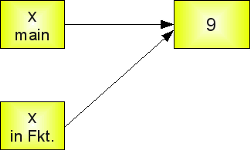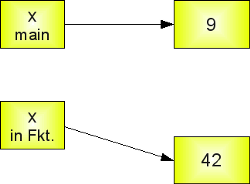## Parameter Passing

### "call by value" and "call by name"The most common evaluation strategy when passing arguments to a function has been call by value and call by reference:

• Call by Value
The most common strategy is the call-by-value evaluation, sometimes also called pass-by-value. This strategy is used in C and C++, for example. In call-by-value, the argument expression is evaluated, and the result of this evaluation is bound to the corresponding variable in the function. So, if the expression is a variable, a local copy of its value will be used, i.e. the variable in the caller's scope will be unchanged when the function returns.
• Call by Reference
In call-by-reference evaluation, which is also known as pass-by-reference, a function gets an implicit reference to the argument, rather than a copy of its value. As a consequence, the function can modify the argument, i.e. the value of the variable in the caller's scope can be changed. The advantage of call-by-reference consists in the advantage of greater time- and space-efficiency, because arguments do not need to be copied. On the other hand this harbours the disadvantage that variables can be "accidentally" changed in a function call. So special care has to be taken to "protect" the values, which shouldn't be changed.
Many programming language support call-by-reference, like C or C++, but Perl uses it as default.
In ALGOL 60 and COBOL has been known a different concept, which was called call-by-name, which isn't used anymore in modern languages.There are books which call the strategy of Python call-by-value and others call it call-by-reference. You may ask yourself, what is right.

Humpty Dumpty supplies the explanation:

--- "When I use a word," Humpty Dumpty said, in a rather a scornful tone, "it means just what I choose it to mean - neither more nor less."
--- "The question is," said Alice, "whether you can make words mean so many different things."
--- "The question is," said Humpty Dumpty, "which is to be master - that's all."
Lewis Carroll, Through the Looking-Glass

To come back to our initial question what is used in Python: The authors who call the mechanism call-by-value and those who call it call-by-reference are stretching the definitions until they fit.
Correctly speaking, Python uses a mechanism, which is known as "Call-by-Object", sometimes also called "Call by Object Reference" or "Call by Sharing".If you pass immutable arguments like integers, strings or tuples to a function, the passing acts like call-by-value. The object reference is passed to the function parameters. They can't be changed within the function, because they can't be changed at all, i.e. they are immutable. It's different, if we pass mutable arguments. They are also passed by object reference, but they can be changed in place in the function. If we pass a list to a function, we have to consider two cases: Elements of a list can be changed in place, i.e. the list will be changed even in the caller's scope. If a new list is assigned to the name, the old list will not be affected, i.e. the list in the caller's scope will remain untouched.

First, let's have a look at the integer variables. The parameter inside of the function remains a reference to the arguments variable, as long as the parameter is not changed. As soon as a new value will be assigned to it, Python creates a separate local variable. The caller's variable will not be changed this way:
def ref_demo(x):
print "x=",x," id=",id(x)
x=42
print "x=",x," id=",id(x)In the example above, we used the id() function, which takes an object as a parameter. id(obj) returns the "identity" of the object "obj". This identity, the return value of the function, is an integer which is unique and constant for this object during its lifetime. Two different objects with non-overlapping lifetimes may have the same id() value.

If you call the function ref_demo() - like we do in the green block further down - we can check with the id() function what happens to x. We can see that in the main scope, x has the identity 41902552. In the first print statement of the ref_demo() function, the x from the main scope is used, because we can see that we get the same identity. After we have assigned the value 42 to x, x gets a new identity 41903752, i.e. a separate memory location from the global x. So, when we are back in the main scope x has still the original value 9.

This means that Python initially behaves like call-by-reference, but as soon as we are changing the value of such a variable, Python "switches" to call-by-value.
>>> x = 9
>>> id(x)
41902552
>>> ref_demo(x)
x= 9  id= 41902552
x= 42  id= 41903752
>>> id(x)
41902552
>>>


### Side effects

A function is said to have a side effect if, in addition to producing a value, it modifies the caller's environment in other ways. For example, a function might modify a global or static variable, modify one of its arguments, raise an exception, write data to a display or file and so on.

In many cases these side effects are wanted, i.e. they are part of the functions specification. But in other cases, they are not wanted , they are hidden side effects. In this chapter we are only interested in the side effects, which change global variables, which have been passed as arguments to a function.
Let's assume, we are passing a list to a function. We expect that the function is not changing this list. First let's have a look at a function which has no side effects. As a new list is assigned to the parameter list in func1(), a new memory location is created for list and list becomes a local variable.
>>> def func1(list):
...     print list
...     list = [47,11]
...     print list
...
>>> fib = [0,1,1,2,3,5,8]
>>> func1(fib)
[0, 1, 1, 2, 3, 5, 8]
[47, 11]
>>> print fib
[0, 1, 1, 2, 3, 5, 8]
>>>

This changes drastically, if we include something in the list by using +=. To show this, we have a different function func2() in the following example:
>>> def func2(list):
...     print list
...     list += [47,11]
...     print list
...
>>> fib = [0,1,1,2,3,5,8]
>>> func2(fib)
[0, 1, 1, 2, 3, 5, 8]
[0, 1, 1, 2, 3, 5, 8, 47, 11]
>>> print fib
[0, 1, 1, 2, 3, 5, 8, 47, 11]
>>>

The user of the function can prevent this by passing a copy to the function. In this case a shallow copy is sufficient:
>>> def func2(list):
...     print list
...     list += [47,11]
...     print list
...
>>> fib = [0,1,1,2,3,5,8]
>>> func2(fib[:])
[0, 1, 1, 2, 3, 5, 8]
[0, 1, 1, 2, 3, 5, 8, 47, 11]
>>> print fib
[0, 1, 1, 2, 3, 5, 8]
>>>


### Command Line Arguments

It's possible to write Python scripts using command line arguments. If you call a Python script from a shell, the arguments are placed after the script name. The arguments are separated by spaces. Inside of the script these arguments are accessible through the list variable sys.argv. The name of the script is included in this list sys.argv. sys.argv contains the first parameter, sys.argv the second and so on.
The following script (arguments.py) prints all arguments:
 # Module sys has to be imported:
import sys

# Iteration over all arguments:
for eachArg in sys.argv:
print eachArg

Example call to this script:
python argumente.py python course for beginners

This call creates the following output:
argumente.py
python
course
for
beginners


### Variable Length of Parameters

We will introduce now functions, which can take an arbitrary number of arguments. Those who have some programming background in C or C++ know this from the varargs feature of these languages. The asterisk "*" is used in Python to define a variable number of arguments. The asterisk character has to precede a variable identifier in the parameter list.
>>> def varpafu(*x): print(x)
...
>>> varpafu()
()
>>> varpafu(34,"Do you like Python?", "Of course")
(34, 'Do you like Python?', 'Of course')
>>>

We learn from the previous example that the arguments passed to the function call of varpafu() are collected in a tuple, which can be accessed as a "normal" variable x within the body of the function. If the function is called without any arguments, the value of x is an empty tuple.

Sometimes, it's necessary to use positional parameters followed by an arbitrary number of parameters in a function definition. This is possible, but the positional parameters always have to precede the arbitrary parameters. In the following example, we have a positional parameter "city", - the main location, - which always have to be given, followed by an arbitrary number of other locations:
>>> def locations(city, *other_cities): print(city, other_cities)
...
>>> locations("Paris")
('Paris', ())
>>> locations("Paris", "Strasbourg", "Lyon", "Dijon", "Bordeaux", "Marseille")
('Paris', ('Strasbourg', 'Lyon', 'Dijon', 'Bordeaux', 'Marseille'))
>>>


#### Exercise

Write a function which calculates the arithmetic mean of a variable number of values.

#### Solution

def arithmetic_mean(x, *l):
""" The function calculates the arithmetic mean of a non-empty
arbitrary number of numbers """
sum = x
for i in l:
sum += i

return sum / (1.0 + len(l))

You might ask yourself, why we used both a positional parameter "x" and the variable parameter "*l" in our function definition. We could have only used *l to contain all our numbers. The idea is that we wanted to enforce that we always have a non-empty list of numbers. This is necessary to prevent a division by zero error, because the average of a an empty list of numbers is not defined.

In the following interactive Python session, we can learn how to use this function. We assume that the function arithmetic_mean is saved in a file called statistics.py.
>>> from statistics import arithmetic_mean
>>> arithmetic_mean(4,7,9)
6.666666666666667
>>> arithmetic_mean(4,7,9,45,-3.7,99)
26.71666666666667

This works fine, but there is a catch. What if somebody wants to call the function with a list, instead of a variable number of numbers, as we have shown above? We can see in the following that we raise an error, as most hopefully, you might expect:
>>> l = [4,7,9,45,-3.7,99]
>>> arithmetic_mean(l)
Traceback (most recent call last):
File "<stdin>", line 1, in <module>
File "statistics.py", line 8, in arithmetic_mean
return sum / (1.0 + len(l))
TypeError: unsupported operand type(s) for /: 'list' and 'float'

The rescue is using another asterisk:
>>> arithmetic_mean(*l)
26.71666666666667
>>>


### * in Function Calls

A * can appear in function calls as well, as we have just seen in the previous exercise: The semantics is in this case "inverse" to a star in a function definition. An argument will be unpacked and not packed. In other words, the elements of the list or tuple are singularized:
>>> def f(x,y,z):
...     print(x,y,z)
...
>>> p = (47,11,12)
>>> f(*p)
(47, 11, 12)

There is hardly any need to mention that this way of calling our function is more comfortable the following one:
>>> f(p,p,p)
(47, 11, 12)
>>>

Additionally to being less comfortable, the previous call (f(p,p,p)) doesn't work in the general case, i.e. lists of unknown lengths. "Unknown" mean, that the length is only known at runtime and not when we are writing the script.

### Arbitrary Keyword Parameters

There is also a mechanism for an arbitrary number of keyword parameters. To do this, we use the double asterisk "**" notation:
>>> def f(**args):
...     print(args)
...
>>> f()
{}
>>> f(de="German",en="English",fr="French")
{'fr': 'French', 'de': 'German', 'en': 'English'}
>>>


### Double Asterisk in Function Calls

The following example demonstrates the usage of ** in a function call:
>>> def f(a,b,x,y):
...     print(a,b,x,y)
...
>>> d = {'a':'append', 'b':'block','x':'extract','y':'yes'}
>>> f(**d)
('append', 'block', 'extract', 'yes')

and now in combination with *:
>>> t = (47,11)
>>> d = {'x':'extract','y':'yes'}
>>> f(*t, **d)
(47, 11, 'extract', 'yes')
>>>